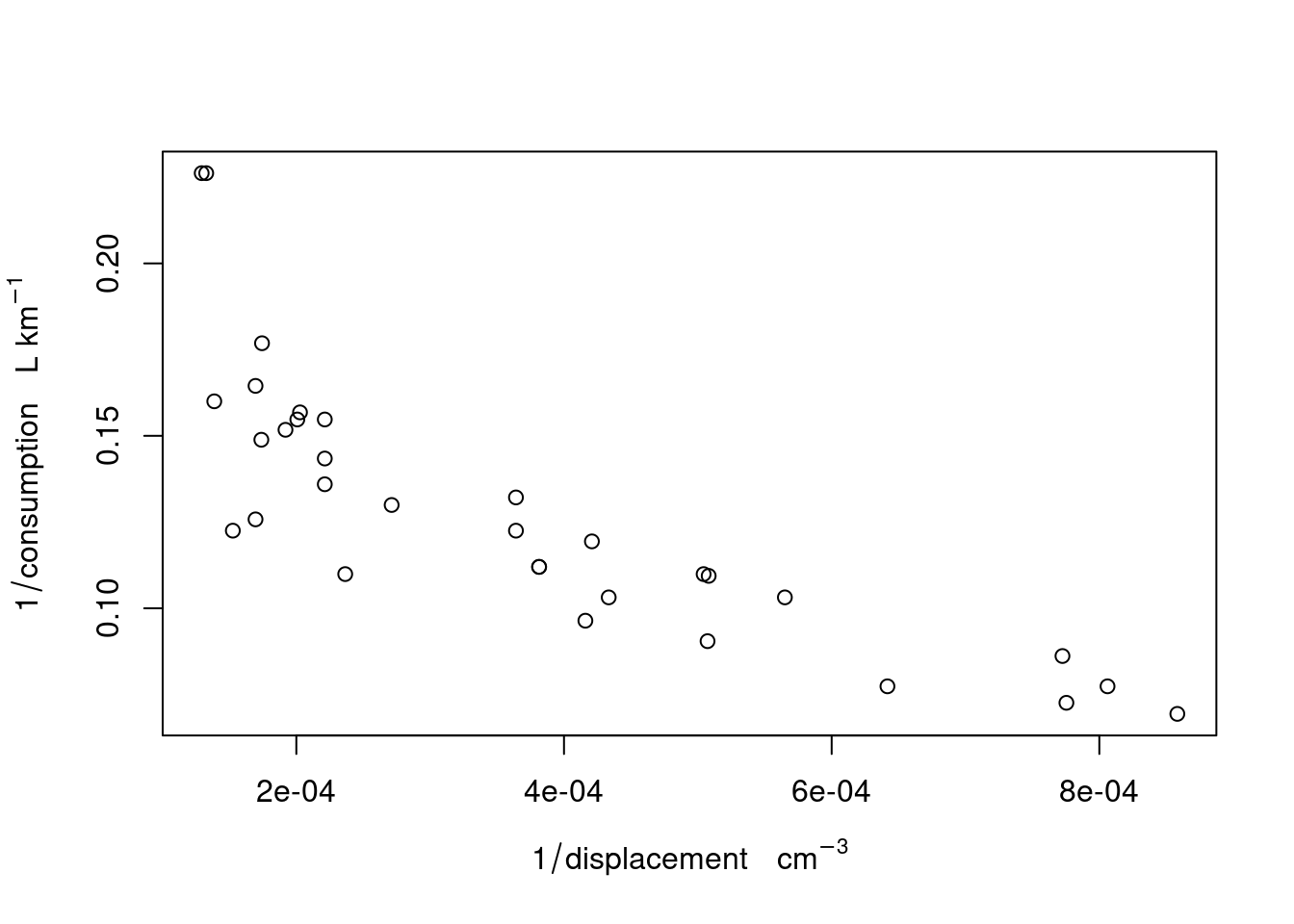R has little support for physical measurement units. The exception is formed by time differences: time differences objects of class difftime have a units attribute that can be modified:

t1 = Sys.time()
t2 = t1 + 3600
d = t2 - t1
class(d)
##  "difftime"
units(d)
##  "hours"
d
## Time difference of 1 hours
units(d) = "secs"
d
## Time difference of 3600 secs

We see here that the units method is used to retrieve and modify the unit of time differences.

The units package generalizes this idea to other physical units, building upon the udunits2 R package, which in turn is build upon the udunits2 C library. The udunits2 library provides the following operations:

• validating whether an expression, such as m/s is a valid physical unit
• verifying whether two units such as m/s and km/h are convertible
• converting values between two convertible units
• providing names and symbols for specific units
• handle different character encodings (utf8, ascii, iso-8859-1 and latin1)

The units R package uses R package udunits2 to extend R with functionality for manipulating numeric vectors that have physical measurement units associated with them, in a similar way as difftime objects behave.

Setting units, unit conversion

We can set units to numerical values by set_units:

library(units)
(a <- set_units(runif(10),  m/s))
## Units: [m/s]
##   0.9641523 0.7180470 0.6880640 0.8542836 0.5427593 0.6925464 0.9029404
##   0.3737604 0.8441793 0.2801690

the result, e.g.

set_units(10, m/s)
## 10 [m/s]

literally means “10 times 1 m divided by 1 s”. In writing, the “1” values are omitted, and the multiplication is implicit.

Unit conversion

When conversion is meaningful, such as hours to seconds or meters to kilometers, conversion can be done explicitly by setting the units of a vector

b = a
units(b) <- make_units(km/h)
b
## Units: [km/h]
##   3.470948 2.584969 2.477030 3.075421 1.953933 2.493167 3.250586 1.345538
##   3.039045 1.008608

Basic manipulations

Arithmetic operations

Arithmetic operations verify units, and create new ones

a + a
## Units: [m/s]
##   1.9283046 1.4360941 1.3761279 1.7085671 1.0855185 1.3850929 1.8058809
##   0.7475208 1.6883585 0.5603380
a * a
## Units: [m^2/s^2]
##   0.92958968 0.51559154 0.47343200 0.72980039 0.29458761 0.47962056
##   0.81530142 0.13969685 0.71263862 0.07849467
a ^ 2
## Units: [m^2/s^2]
##   0.92958968 0.51559154 0.47343200 0.72980039 0.29458761 0.47962056
##   0.81530142 0.13969685 0.71263862 0.07849467
a ** -2
## Units: [s^2/m^2]
##    1.075743  1.939520  2.112236  1.370238  3.394576  2.084982  1.226540
##    7.158358  1.403236 12.739719

and convert to the units of the first argument if necessary:

a + b # m/s + km/h -> m/s
## Units: [m/s]
##   1.9283046 1.4360941 1.3761279 1.7085671 1.0855185 1.3850929 1.8058809
##   0.7475208 1.6883585 0.5603380

Currently, powers are only supported for integer powers, so using a ** 2.5 would result in an error.

Unit simplification

There are some basic simplification of units:

t <- make_units(s)
a * t
## Units: [m]
##   0.9641523 0.7180470 0.6880640 0.8542836 0.5427593 0.6925464 0.9029404
##   0.3737604 0.8441793 0.2801690

which also work when units need to be converted before they can be simplified:

t <- make_units(min)
a * t
## Units: [m]
##   57.84914 43.08282 41.28384 51.25701 32.56556 41.55279 54.17643 22.42563
##   50.65076 16.81014

Simplification to unit-less values gives the “1” as unit:

m <- make_units(m)
a * t / m
## Units: 
##   57.84914 43.08282 41.28384 51.25701 32.56556 41.55279 54.17643 22.42563
##   50.65076 16.81014

Allowed operations that require convertible units are +, -, ==, !=, <, >, <=, >=. Operations that lead to new units are *, /, and the power operations ** and ^.

Mathematical functions

Mathematical operations allowed are: abs, sign, floor, ceiling, trunc, round, signif, log, cumsum, cummax, cummin.

signif(a ** 2 / 3, 3)
## Units: [m^2/s^2]
##   0.3100 0.1720 0.1580 0.2430 0.0982 0.1600 0.2720 0.0466 0.2380 0.0262
cumsum(a)
## Units: [m/s]
##   0.9641523 1.6821993 2.3702633 3.2245468 3.7673061 4.4598525 5.3627930
##   5.7365534 6.5807326 6.8609016
log(a) # base defaults to exp(1)
## Units: [(ln(re 1 m.s-1))]
##   -0.0365060 -0.3312202 -0.3738735 -0.1574921 -0.6110894 -0.3673800
##   -0.1020987 -0.9841403 -0.1693904 -1.2723623
log(a, base = 10)
## Units: [(lg(re 1 m.s-1))]
##   -0.01585435 -0.14384711 -0.16237119 -0.06839796 -0.26539276 -0.15955110
##   -0.04434090 -0.42740669 -0.07356532 -0.55257992
log(a, base = 2)
## Units: [(lb(re 1 m.s-1))]
##   -0.05266702 -0.47784975 -0.53938543 -0.22721309 -0.88161566 -0.53001729
##   -0.14729729 -1.41981430 -0.24437872 -1.83563078

Summary functions

Summary functions sum, min, max, and range are allowed:

sum(a)
## 6.860902 [m/s]
min(a)
## 0.280169 [m/s]
max(a)
## 0.9641523 [m/s]
range(a)
## Units: [m/s]
##  0.2801690 0.9641523
make_units(min(m/s, km/h)) # converts to first unit:
## 0.2777778 [m/s]

Printing

Following difftime, printing behaves differently for length-one vectors:

a
## Units: [m/s]
##   0.9641523 0.7180470 0.6880640 0.8542836 0.5427593 0.6925464 0.9029404
##   0.3737604 0.8441793 0.2801690
a
## 0.9641523 [m/s]

Subsetting

The usual subsetting rules work:

a[2:5]
## Units: [m/s]
##  0.7180470 0.6880640 0.8542836 0.5427593
a[-(1:9)]
## 0.280169 [m/s]

Concatenation

c(a,a)
## Units: [m/s]
##   0.9641523 0.7180470 0.6880640 0.8542836 0.5427593 0.6925464 0.9029404
##   0.3737604 0.8441793 0.2801690 0.9641523 0.7180470 0.6880640 0.8542836
##  0.5427593 0.6925464 0.9029404 0.3737604 0.8441793 0.2801690

concatenation converts to the units of the first argument, if necessary:

c(a,b) # m/s, km/h -> m/s
## Units: [m/s]
##   0.9641523 0.7180470 0.6880640 0.8542836 0.5427593 0.6925464 0.9029404
##   0.3737604 0.8441793 0.2801690 0.9641523 0.7180470 0.6880640 0.8542836
##  0.5427593 0.6925464 0.9029404 0.3737604 0.8441793 0.2801690
c(b,a) # km/h, m/s -> km/h
## Units: [km/h]
##   3.470948 2.584969 2.477030 3.075421 1.953933 2.493167 3.250586 1.345538
##   3.039045 1.008608 3.470948 2.584969 2.477030 3.075421 1.953933 2.493167
##  3.250586 1.345538 3.039045 1.008608

Conversion to/from difftime

From difftime to units:

t1 = Sys.time()
t2 = t1 + 3600
d = t2 - t1
(du = as_units(d))
## 1 [h]

vice versa:

(dt = as_difftime(du))
## Time difference of 1 hours
class(dt)
##  "difftime"

units in matrix objects

set_units(matrix(1:4,2,2), m/s)
## Units: [m/s]
##      [,1] [,2]
## [1,]    1    3
## [2,]    2    4
set_units(matrix(1:4,2,2), m/s * m/s)
## Units: [m^2/s^2]
##      [,1] [,2]
## [1,]    1    3
## [2,]    2    4

but

set_units(matrix(1:4,2,2), m/s) %*% set_units(4:3, m/s)
##      [,1]
## [1,]   13
## [2,]   20

strips units.

units objects in data.frames

units in data.frame objects are printed, but do not appear in summary:.

set.seed(131)
d <- data.frame(x = runif(4),
y = set_units(runif(4), s),
z = set_units(1:4, m/s))
d
##           x             y       z
## 1 0.2064370 0.8463468 [s] 1 [m/s]
## 2 0.1249422 0.5292048 [s] 2 [m/s]
## 3 0.2932732 0.5186254 [s] 3 [m/s]
## 4 0.3757797 0.2378545 [s] 4 [m/s]
summary(d)
##        x                y                z
##  Min.   :0.1249   Min.   :0.2379   Min.   :1.00
##  1st Qu.:0.1861   1st Qu.:0.4484   1st Qu.:1.75
##  Median :0.2499   Median :0.5239   Median :2.50
##  Mean   :0.2501   Mean   :0.5330   Mean   :2.50
##  3rd Qu.:0.3139   3rd Qu.:0.6085   3rd Qu.:3.25
##  Max.   :0.3758   Max.   :0.8463   Max.   :4.00
d$yz = with(d, y * z) d ## x y z yz ## 1 0.2064370 0.8463468 [s] 1 [m/s] 0.8463468 [m] ## 2 0.1249422 0.5292048 [s] 2 [m/s] 1.0584095 [m] ## 3 0.2932732 0.5186254 [s] 3 [m/s] 1.5558761 [m] ## 4 0.3757797 0.2378545 [s] 4 [m/s] 0.9514180 [m] d[1, "yz"] ## 0.8463468 [m] formatting Units are often written in the form m2 s-1, for square meter per second. This can be defined as unit, and also parsed by as_units: (x = 1:10 * as_units("m2 s-1")) ## Units: [m^2/s] ##  1 2 3 4 5 6 7 8 9 10 udunits understands such string, and can convert them y = 1:10 * make_units(m^2/s) x + y ## Units: [m^2/s] ##  2 4 6 8 10 12 14 16 18 20 Printing units in this form is done by deparse_unit(x) ##  "m2 s-1" plotting Base scatter plots and histograms support automatic unit placement in axis labels. In the following example we first convert to SI units. (Unit in needs a bit special treatment, because in is a reserved word in R.) mar = par("mar") + c(0, .3, 0, 0) displacement = mtcars$disp * as_units("in")^3
units(displacement) = make_units(cm^3)
weight = mtcars$wt * 1000 * make_units(lb) units(weight) = make_units(kg) par(mar = mar) plot(weight, displacement)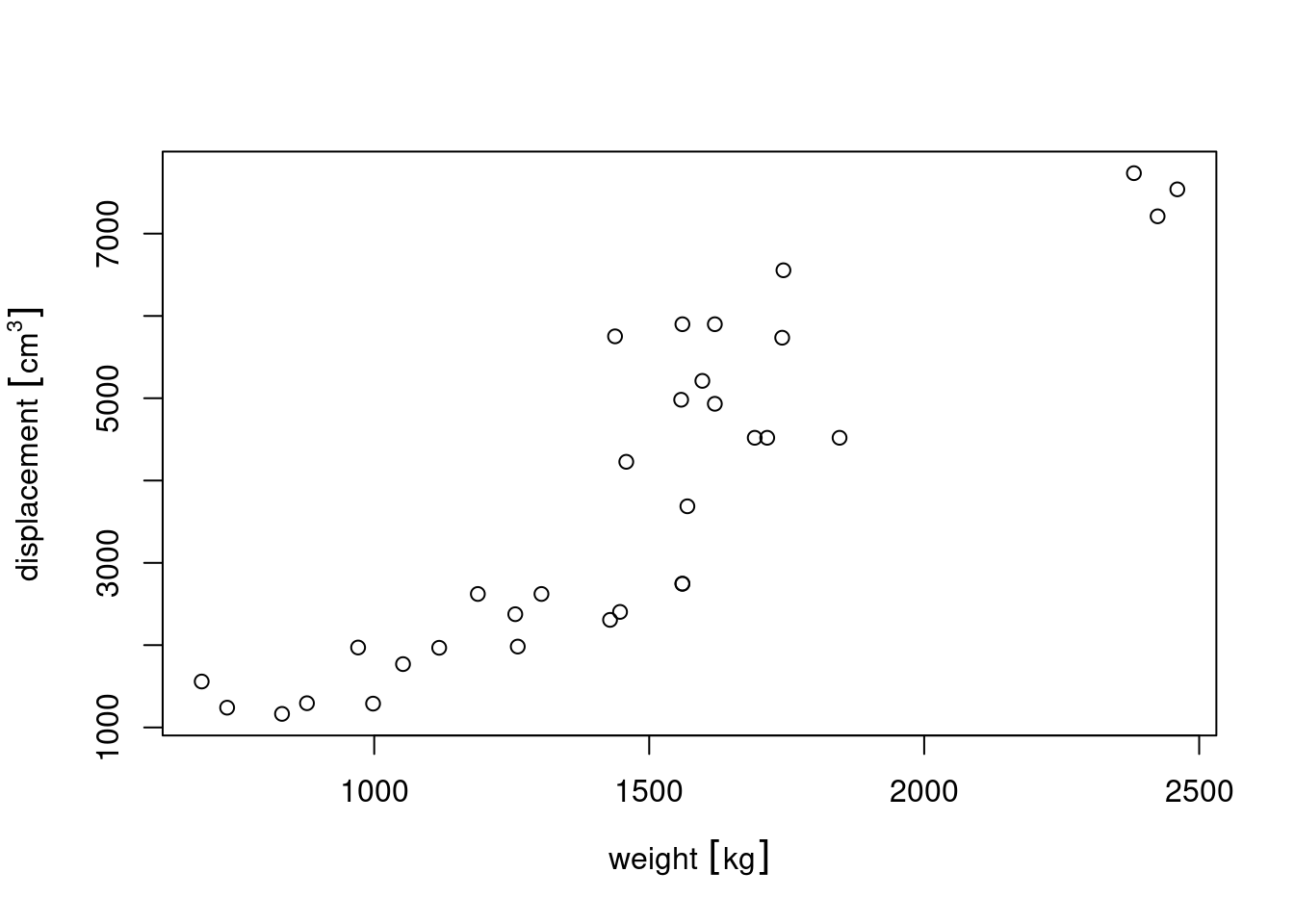We can change grouping symbols from [ ] into ( ): units_options(group = c("(", ")") ) # parenthesis instead of square brackets par(mar = mar) plot(weight, displacement)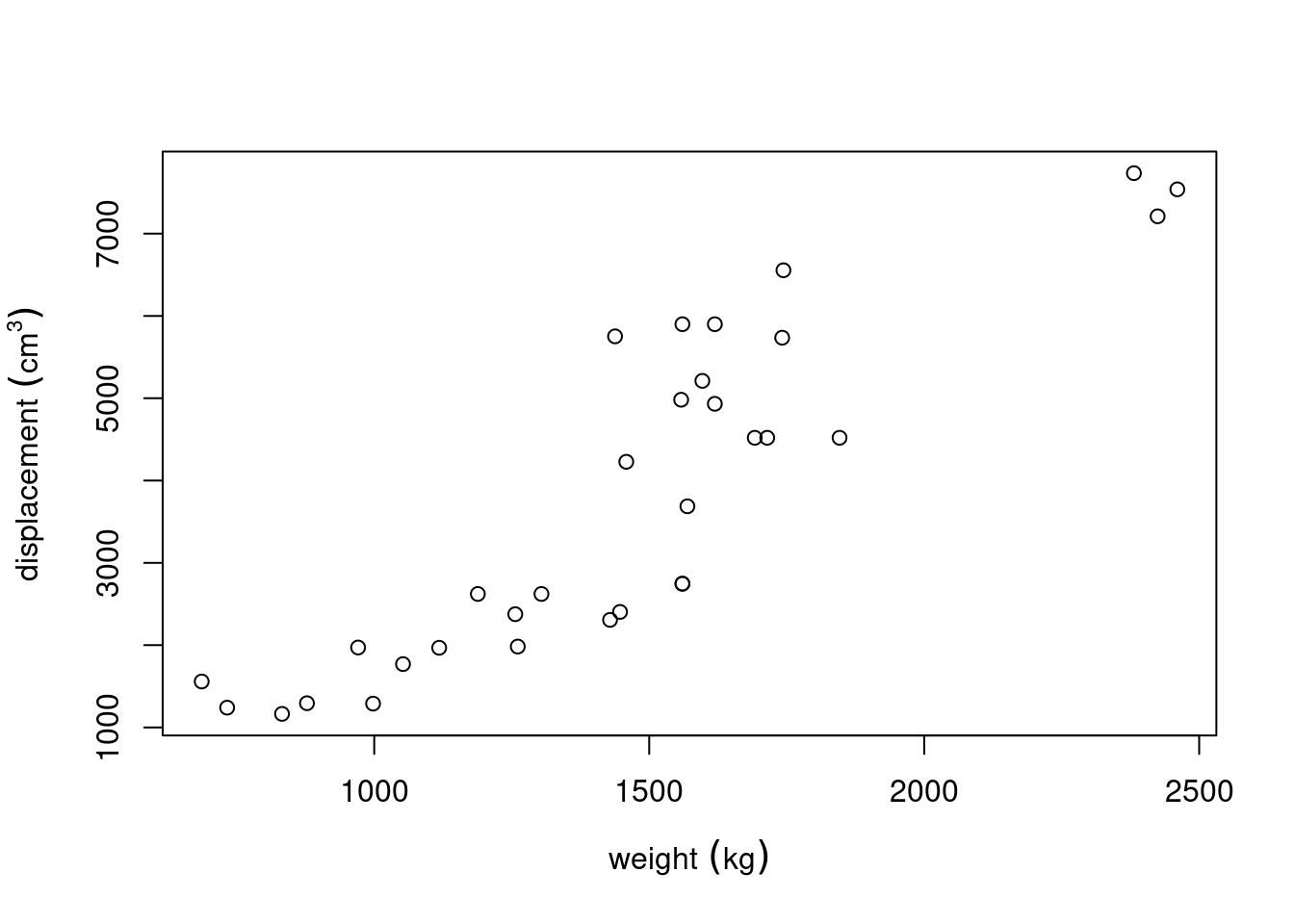We can also remove grouping symbols, increase space between variable name and unit by: units_options(sep = c("~~~", "~"), group = c("", "")) # no brackets; extra space par(mar = mar) plot(weight, displacement)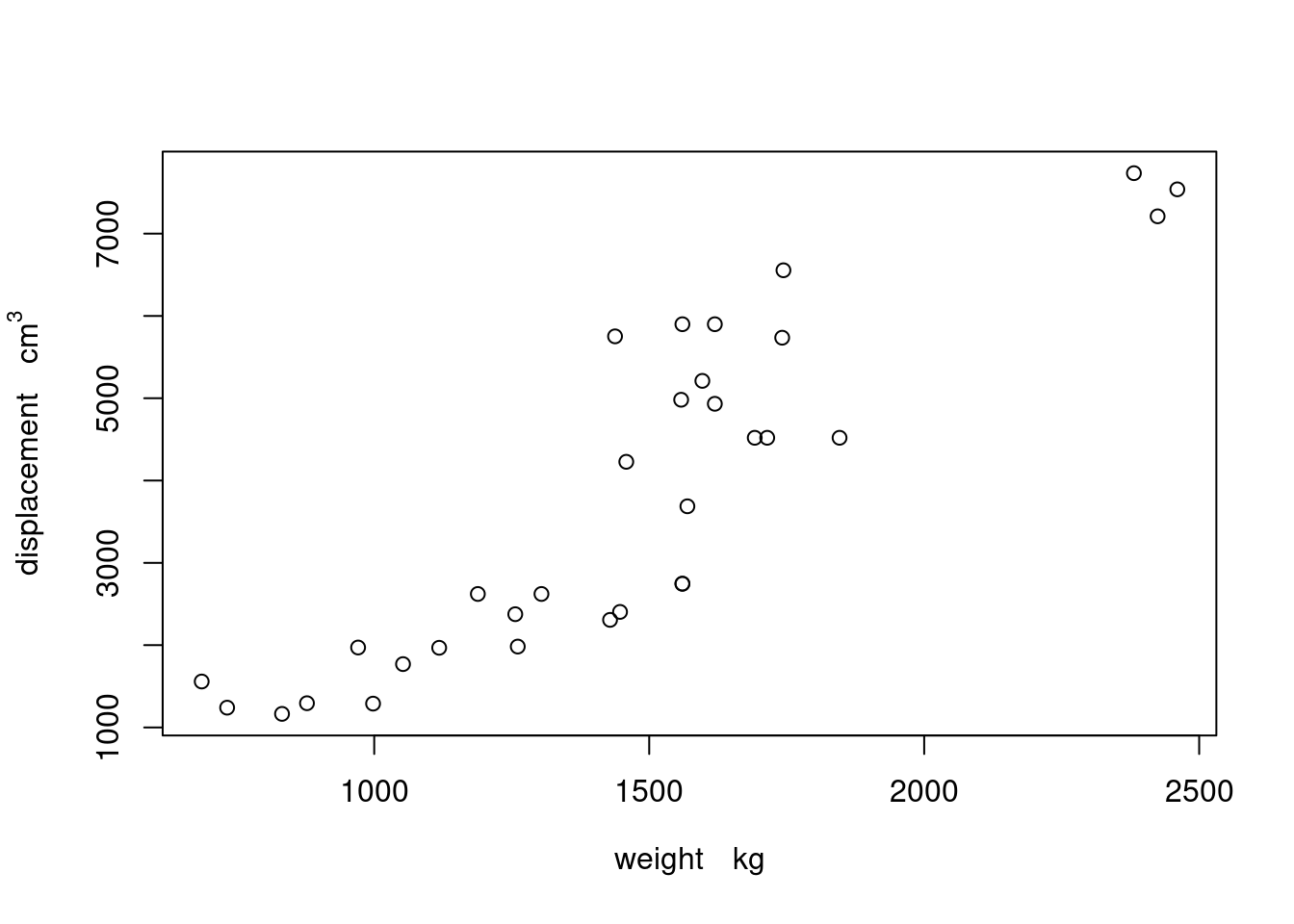More complex units can be plotted either with negative powers, or as divisions, by modifying one of units’s global options using units_options: gallon = as_units("gallon") consumption = mtcars$mpg * make_units(mi/gallon)
units(consumption) = make_units(km/l)
par(mar = mar)
plot(displacement, consumption) # division in consumption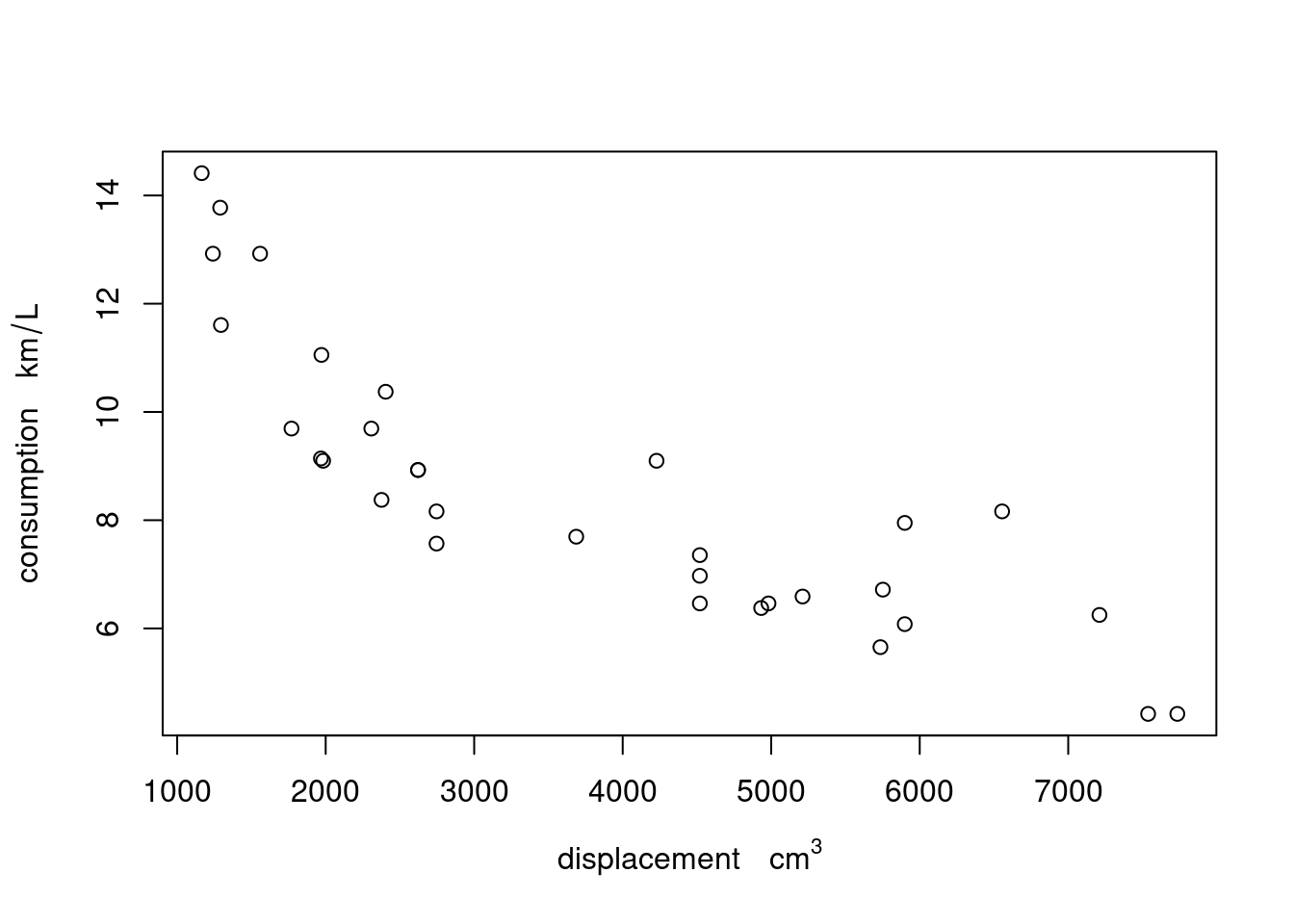units_options(negative_power = TRUE) # division becomes ^-1
plot(displacement, consumption) # division in consumption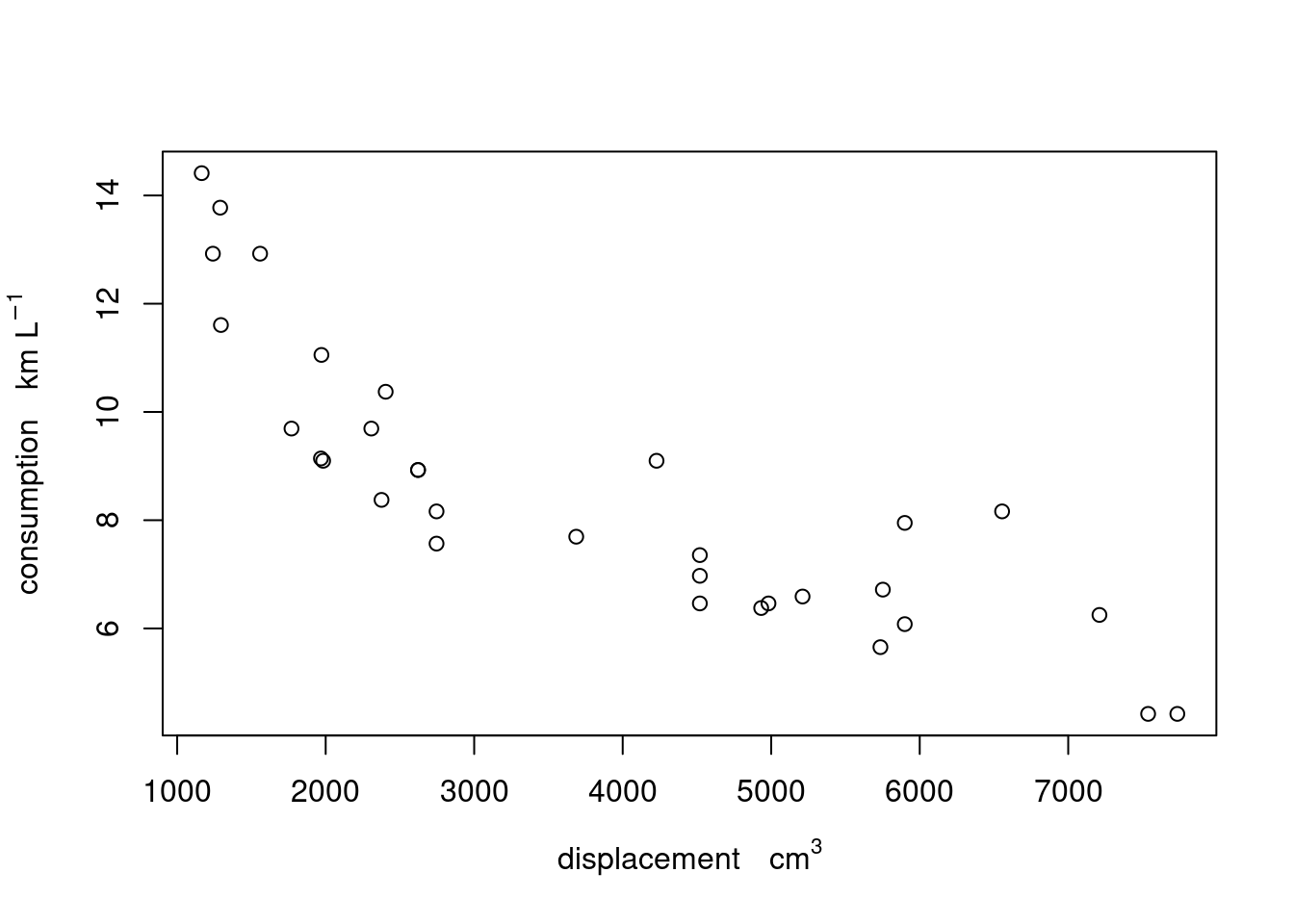As usual, units modify automatically in expressions:

units_options(negative_power = TRUE) # division becomes ^-1
par(mar = mar)
plot(displacement, consumption)plot(1/displacement, 1/consumption)